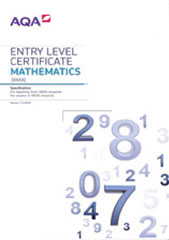# Level descriptions

These descriptions give a general indication of the standards of achievement at each level. These descriptions should be interpreted in relation to the content outlined in the Subject content part of this specification; they are not designed to define that content.

## Entry Level

### Entry Level 1

Students use mathematics as an integral part of classroom activities. They represent their work with objects or pictures and discuss it. They recognise and use a simple pattern or relationship.

Students count, order, add and subtract numbers when solving problems involving up to 20 objects. They read and write the numbers involved. They understand that subtraction is the inverse of addition. They work out or identify half of a quantity.

Students understand the money system of coins and notes. They know the days of the week and understand times on the hour or half hour.

When working with 2D and 3D shapes, students use everyday language to describe properties and positions. They measure and order objects using direct comparison, and order events.

Students sort objects and classify them, demonstrating the criterion they have used.

Students can construct and interpret simple lists and graphs.

### Entry Level 2

Students select the mathematics they use in some classroom activities. They discuss their work using mathematical language and are beginning to represent it using symbols and simple diagrams. They explain why an answer is correct.

Students count sets of objects reliably and use mental recall of addition and subtraction facts to 20. They begin to understand the place value of each digit in a number and use this to order numbers up to 100. They choose the appropriate operation when solving addition, subtraction and multiplication problems. They use mental calculation strategies to solve number problems involving money and measures. They recognise sequences of numbers, including odd and even numbers. They use simple fractions that are one part of a whole.

Students convert from pounds to pence and vice versa. They add amounts of money and give change. They can read times to the nearest five minutes on an analogue clock and can read anytime on a digital clock.

Students use mathematical names for common 2D and 3D shapes and describe their properties, including numbers of edges, faces and vertices. They distinguish between straight and turning movements, understand angle as a measurement of turn, and recognise right angles in turns. They begin to use standard units to measure length and weight.

Students sort objects and classify them using more than one criterion. When they have gathered information, they record results in simple lists, tables and block graphs in order to communicate their findings.

### Entry Level 3

Students try different approaches and find ways of overcoming difficulties that arise when they are solving problems. They are beginning to organise their work and check results. Students discuss their mathematical work and are beginning to explain their thinking. They use and interpret mathematical symbols and diagrams. Students show that they understand a general statement by finding particular examples that match it.

Students show understanding of place value in numbers up to 1,000 and use this to make approximations. They begin to use decimal notation and to recognise negative numbers, in contexts such as temperature. They add and subtract numbers with two digits mentally and numbers with three digits using written methods. They use mental recall of the 2, 3, 4, 5, 8 and 10 multiplication tables and derive the associated division facts. They solve whole number problems involving multiplication or division, including those that give rise to remainders. They use simple fractions that are several parts of a whole and recognise when two simple fractions are equivalent.

Students solve multi-step problems involving money and give answers in correct notation.

Students classify 2D and 3D shapes in various ways using mathematical properties such as reflective symmetry for 2D shapes. They use standard metric units of length, capacity and weight and standard units of time, in a range of contexts.

Students extract and interpret information presented in simple tables and lists. They construct bar charts and pictograms, where the symbol represents more than one item to communicate information and they interpret information presented to them in these forms.